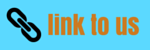# System of particles and Collision

## (7) Kinetic energy of the system of particles

• Let there are n number of particles in a n particle system and these particles possess some motion. The motion of the i'th particle of this system would depend on the external force Fi acting on it. Let at any time if the velocity of i'th particle be vi then its kinetic energy would be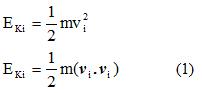• Let ri be the position vector of the i'th particle w.r.t. O and r'i be the position vector of the centre of mass w.r.t. ri ,as shown below in the figure , then
ri=r'i+Rcm                              (2)
where Rcm is the position vector of centre of mass of the system w.r.t. O.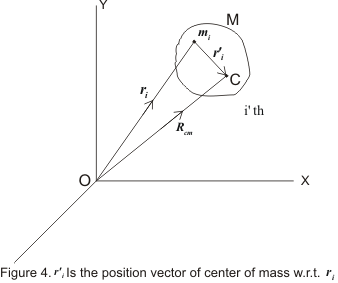• Differentiating equation 2 we get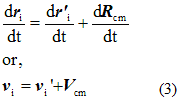where vi is the velocity of i'th particle w.r.t. centre of mass and Vcm is the velocity of centre of mass of system of particle. Putting equation 3 in 1 we get,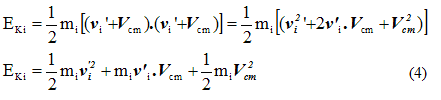• Sum of Kinetic energy of all the particles can be obtained from equation 4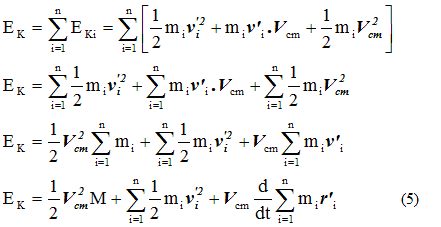• Now last term in above equation which is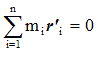would vanish as it defines the null vector because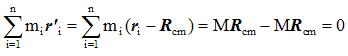• Therefore kinetic energy of the system of particles is,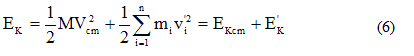where,is the kinetic energy obtained as if all the mass were concentrated at the centre of mass and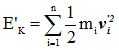is the kinetic energy of the system of particle w.r.t. the centre of mass.
• Hence it is clear from equation 6 that kinetic energy of the system of particles consists of two parts: the kinetic energy obtained as if all the mass were concentrated at the centre of mass plus the kinetic energy of motion about the centre of mass.
• If there were no external force acting on the particle system then the velocity of the centre of mass of the system will remain constant and Kinetic Energy of the system would also remain constant.

## (8) Two particle system and reduced mass

• Two body problems with central forces can always be reduced to the form of one body problems.
• Consider a system made up of two particles. For an observer in any inertial frame of refrence relative motion of these two particles can be represented by the motion of a fictitious particle.
• The mass of this fictetious particle is known as the reduced mass of two particle system.
• Consider a system of two particles of mass m1 and m2 respectively. Let O be the origin of any inertial frame of refrance and r1 and r2 be the position vectors of these particles at any time t w.r.t. origin O as shown bellow in the figure.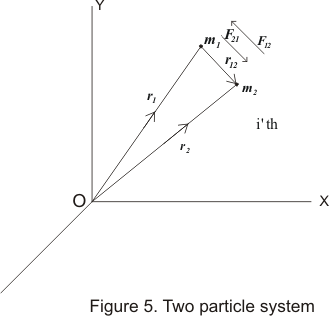• If no external force is acting on the system then the force acting on the system would be equal to mutual interaction between two particles. Let the force acting on m1 due to m2 be F21 and force acting on m2 due to m1 be F12 then equation of motion for particles m1 and m2 would be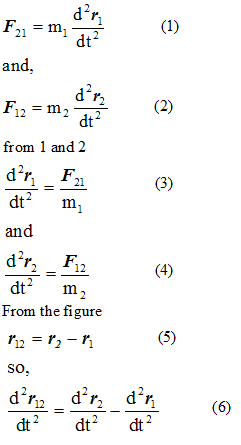putting 3 and 4 in 6 we get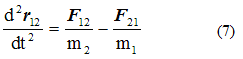but from Newton's first law of motion we have
F21 = -F12
then from equation 7 we have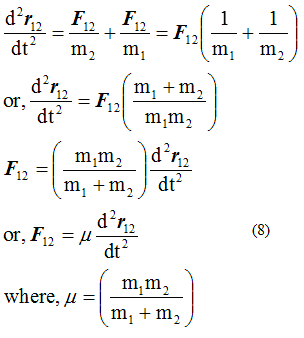is known as reduced mass of the system.
• This equation 8 represents a one body problem , because it is similar to the equation of motion of single particle of mass μ at a vector distance r12 from one of thr two particles, considered as the fixed centre of force.
• Thus original problem involving two particle system has now been reduced to that of one particle system which is easier to solve then original two body problem.
Case 1. m1 << m2
• If the mass of any one particle in two particle system is very very less in comparison to other particle like in earth-satellite system then reduced mass of the system would be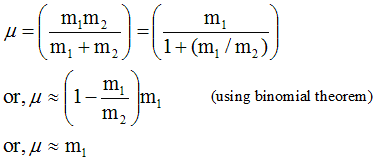• So the reduced mass of the two particle system would be equal to the particle having lesser mass.
Case 2. m1 = m2 = m
• If the masses o8 the particles of a two particle system are same then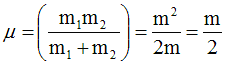• Hence reduced mass of the system would be equal to the one half of the mass of a single particle.

## (9) Linear momentum and principle of conservation of linear momentum

• Product of mass and velocity of any particle is defined as the linear momentum of the particle. It is a vector quantity and its direction is same as the direction of velocity of the particle.
• Linear momentum is represented by p. If m is the mass of the particle moving with velocity v then linear momentum of the particle would be
p=mv
like v , p also depends on the frame of refrance of the observer.
• If in a many particle system m1 , m2 , m3 , . . . . . . . . . . . . . , mn are the masses and v1 , v2 , v3, . . . . . . . . . . . . ., vn are the velocities of the respective particles then total linear momentum of the system would be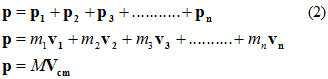where M is the total mass of the system and Vcm is the velocity of centre of mass of the system
• Hence from equation 2 we came to know that total linear momentum of a many particle system is equal to the product of the total mass of the system and velocity of centre of mass of the system.

• Differentiating equation 2 w.r.t. t we get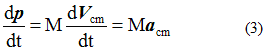but , Macm=Fext which is the external force acting on the system. Therefore,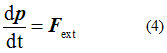like this the rate of change of momentum of a many particle system comes out to be equal to the resultant external force acting on the particle.
• If external force acting on the system is zero then,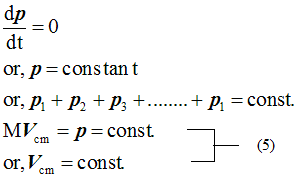• Hence we conclude that when resultant external force acting on any particle is zero then total linear momentum of the system remains constant. This is known as law of conservation of linear momentum.
• Above equation 5 is equivalent to following scalar quantities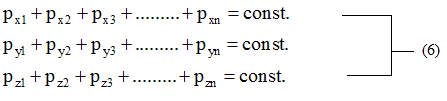Equation 6 shows the total linear momentum of the system in terms of x , y and z co-ordinates and also shows that they remain constant or conserved in absence of any externally applied force.
• The law of conservation of linear momentum is the fundamental and exact law of nature. No violation of it has ever been found. This law has been established on the basis of Newton's law but this law holds true in the situations where Newtonian mechanics fails.

## (10) Centre of mass frame of refrance

• If we attach an inertial frame of refrance with the centre of mass of many particle system then centre of mass in that frame of refrance would be at rest or, Vcm=0 , and such type of refrance frames are known as centre of mass frame of refrance.
• Total linear momentum of a many particle system is zero in centre of mass frame of refrance i.e., pcm=MVcm=0 since Vcm=0.
• Therefore C-refrance frames are also known as zero momentum refrance frames.
• Since in absence of any external force the centre of mass of any system moves with constant velocity in inertial frame of refrance therefore for a many particle system C-rame of refrance is an inertial frame of refrence.
• Refrance frames connected to laboratory are known as L-frame of refrance or lebiratory frame of refrance.